# Capital Asset Pricing Model (CAPM): Formula and Assumptions

CAPM Step-by-Step Calculation and Formula Explanation (with Excel Template)Key Takeaways
• What is the capital asset pricing model (CAPM) used to measure?
• Which components of the CAPM formula have the most impact on the ending output?
• What is the relationship between systematic risk and the expected return on investments?
• What are some drawbacks to beta that receive frequent criticism?

## Cost of Equity Application

To discount the present value (PV) of future cash flows, one of the most important steps is to determine the appropriate discount rate. The discount rate represents the hurdle rate corresponding to the risk profile of the investment (e.g. shares in the target company, proposed project).

Under the specific context of equity investors, the discount rate that pertains to just shareholders is referred to as the “cost of equity” — which is the required rate of return to equity investors that the capital asset pricing model (CAPM) is used to calculate.

Unlevered free cash flows are discounted using the weighted average cost of capital (WACC), whereas levered free cash flows are discounted with the cost of equity. But regardless of the type of cash flow being discounted, the cost of equity serves an integral role in either approach.

## CAPM Formula Components

The cost of equity is most commonly estimated using the CAPM, which links the expected return on a security to its sensitivity to the overall market.

The CAPM comprises three components:

1. Risk-Free Rate (rf): The return received from risk-free investments — most often proxied by the 10-year treasury yield
2. Beta (β): The measurement of the volatility (i.e. systematic risk) of a security compared to the broader market (S&P 500)
3. Equity Risk Premium (rm – rf): The incremental return received from investing in the market (S&P500) above the risk-free rate (rf, as described above)

To explain the fundamental drivers of the CAPM, we’ll briefly discuss each concept in more detail.

## Risk-Free Rate (rf)

Starting off, the risk-free rate should theoretically reflect the yield to maturity of default-free government bonds of equivalent maturity to the duration of each cash flow being discounted.

But due to lack of liquidity in government bonds with the longest maturities (i.e. less trade volume and data sets), the current yield on 10-year US treasury notes has become the standard proxy for the risk-free rate assumption for companies based in the US.

## Beta (β)

Beta measures the systematic risk of a security compared to the broader market (i.e. non-diversifiable risk).

The beta of an asset is calculated as the covariance between expected returns on the asset and the market, divided by the variance of expected returns on the market.

###### Beta/Market Sensitivity Relationship
• β = 0: No Market Sensitivity
• β < 1: Low Market Sensitivity
• β = 1: Same as Market (Neutral)
• β > 1: High Market Sensitivity
• β < 0: Negative Market Sensitivity

For instance, a company with a beta of 1.0 would expect to see returns consistent with the overall stock market returns. So if the market has gone up by 10%, the company should also see a return of 10%.

But if that company were to have a beta of 2.0, it would expect a return of 20% assuming the market had gone up by 10%.

 Systematic Risk Often referred to as market risk, systematic risk is inherent to the entire equities market as opposed to being specific to a particular company or industry Systematic risk is unavoidable and cannot be mitigated through portfolio diversification (e.g. global recessions) Unsystematic Risk Unsystematic risk refers to company-specific (or industry-specific) risk that can actually be reduced through portfolio diversification (e.g. supply chain shutdowns, lawsuit) The benefits of diversification becomes more profound if the portfolio contains investments in different asset classes, industries, and geographies

The common source of criticism related to CAPM is beta, as many view it as a flawed measure of risk.

The standard procedure for estimating the beta of a company is through a regression model that compares the historical market index returns and company-specific returns, in which the slope of the regression line corresponds to the beta of the company’s shares (the calculation is thus “backward looking”).

However, the past performance (and correlation) of a company relative to the market may not be an accurate indicator of future share price performance. The capital structure (debt/equity ratio) of companies also progressively changes over time, which can alter their risk profiles and performance.

Our third input, the equity risk premium, measures the incremental risk (or excess return) of investing in equities over risk-free securities.

Since investing in risky assets such as equities comes with additional risk (i.e. potential for loss of capital), the equity risk premium serves as additional compensation for investors to have an incentive to take on the risk.

The equity risk premium has been around the 4% to 6% range based on historical spreads between the S&P 500 returns over the yields on risk-free government bonds.

Below, the graph of expected returns (y axis) with the beta (x axis) connects the relationships between the three variables.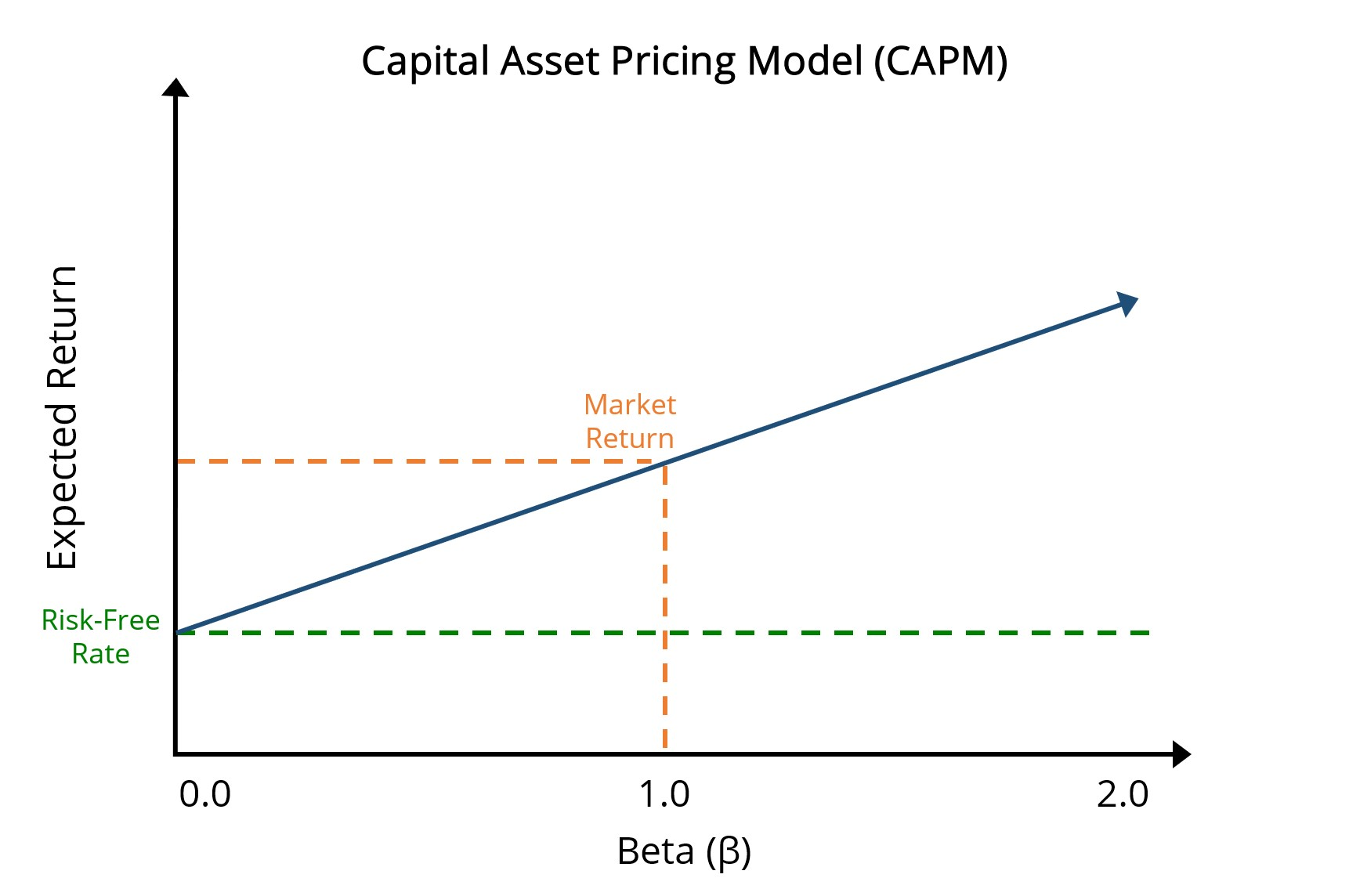Note that the green dotted line represents the risk-free rate, while the orange dotted line depicts the market return (i.e. beta of 1.0). Thus, the difference between the return from the risk-free rate and market return is the equity risk premium.

## CAPM Formula

The CAPM formula is as follows:• Ke → Expected Return on Investment
• rf → Risk-Free Rate
• β → Beta
• (rm – rf) → Equity Risk Premium (ERP)
###### CAPM Simple Example

For a simple example calculation of the cost of equity using CAPM, use the assumptions listed below:

• Risk-Free Rate: 3%
• Beta: 0.8
• Expected Market Return = 10%

Next, by entering this into our formula, we get:

• Cost of Equity (Ke) = 3% + 0.8 (10% – 3%)
• Ke = 8.6%

Now, we’re ready to move to an example calculation of the cost of equity using CAPM. To access the Excel file, just fill out the form below for the download link.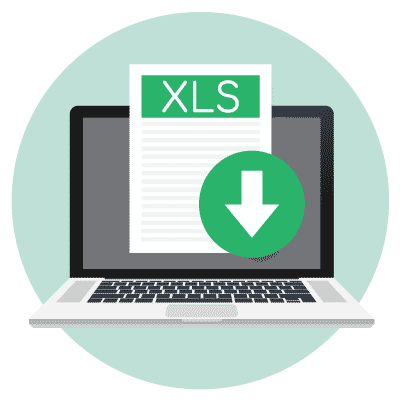Submitting ...

## CAPM Example Calculation Steps

In our hypothetical scenario, we have three companies that each share the following assumptions:

• Risk-Free Rate = 2.5%
• Expected Market Return = 8.0%

Since we’re given the expected return on the market and risk-free rate, we can calculate the equity risk premium for each company using the formula below:

• Equity-Risk Premium (ERP) = 8.0% – 2.5% = 5.5%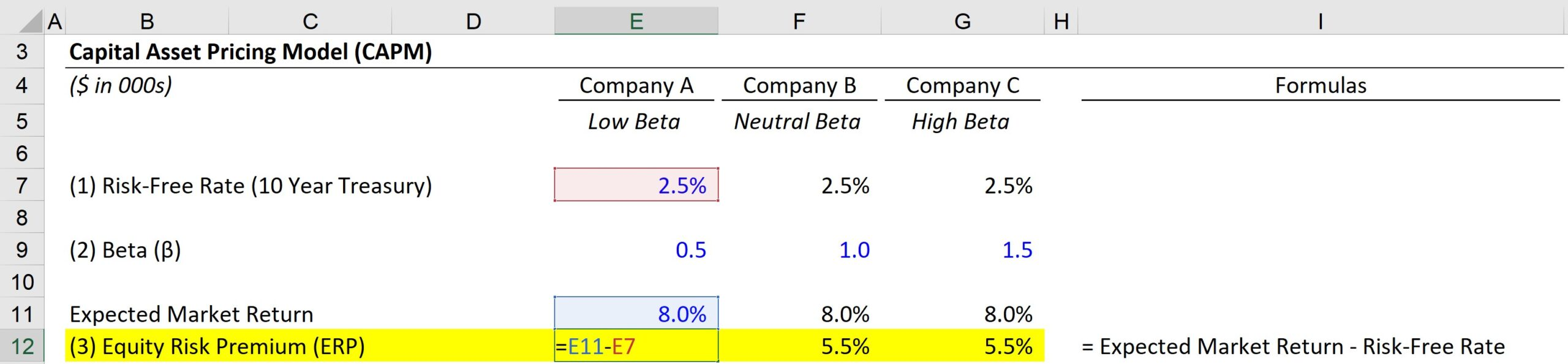The difference in expected returns among the three companies will be attributable to the beta (i.e. systematic risk).

• Company A: 0.5 Beta
• Company B: 1.0 Beta
• Company C: 1.5 Beta

To calculate the cost of equity (Ke), we’ll take the risk-free rate and add it to the product of beta and the equity risk premium, with the ERP calculated as the expected market return minus the risk-free rate.

For example, Company A’s cost of equity can be calculated as:

• Company A: Cost of Equity (Ke) = 2.5% + (0.5 × 5.5%) = 5.3%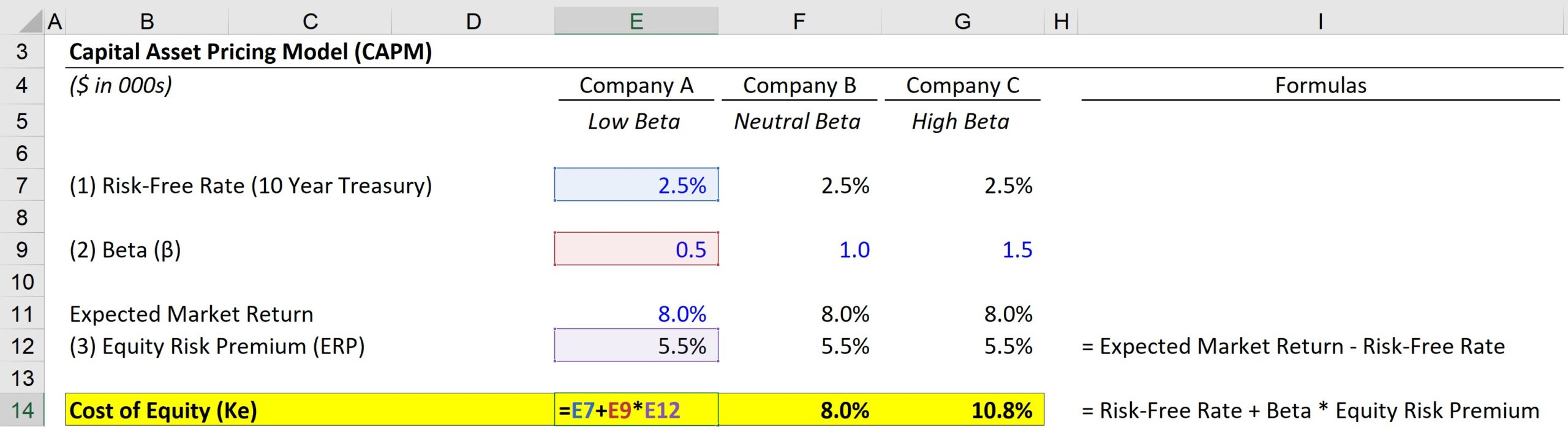Under the provided assumptions, the expected equity returns for the three companies come out to 5.3%, 8.0%, and 10.8%, respectively.

The takeaways from our exercise are the following:

1. The lowest potential returns (and risk) come from the company with the lowest beta
2. The returns from the company with a beta of 1.0 is in line with that of the market
3. The company with the highest potential returns (and risk) has the highest beta

A company with a high beta implies increased risk and higher volatility relative to the overall market (i.e. greater sensitivity to market fluctuations). Therefore, a higher cost of equity would be used by investors to discount the future cash flows generated by the company — which directly leads to a lower valuation, all else being equal.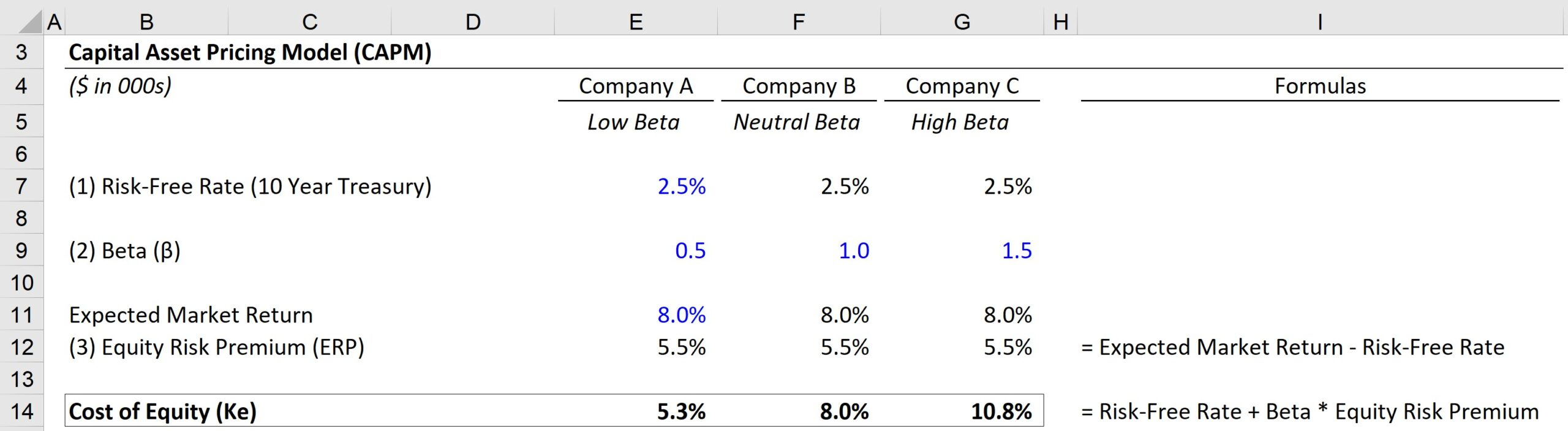Inline FeedbacksLearn Financial Modeling Online

Everything you need to master financial and valuation modeling: 3-Statement Modeling, DCF, Comps, M&A and LBO.

X

The Wall Street Prep Quicklesson Series

7 Free Financial Modeling Lessons

Get instant access to video lessons taught by experienced investment bankers. Learn financial statement modeling, DCF, M&A, LBO, Comps and Excel shortcuts.